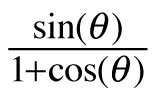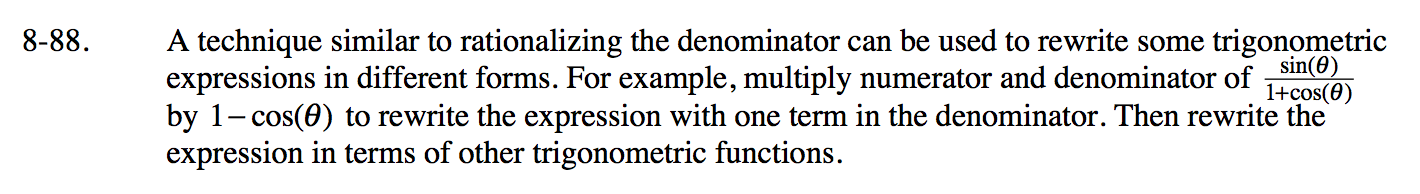### Home > PC3 > Chapter 8 > Lesson 8.3.1 > Problem8-88

8-88.

A technique similar to rationalizing the denominator can be used to rewrite some trigonometric expressions in different forms. For example, multiply numerator and denominator ofby 1 – cos(θ) to rewrite the expression with one term in the denominator. Then rewrite the expression in terms of other trigonometric functions. Homework Help ✎$\frac{\sin(\theta)}{1+\cos(\theta)}\cdot \frac{1-\cos(\theta)}{1-\cos(\theta)}=\frac{\sin(\theta)(1-\cos(\theta)}{1-\cos^{2}(\theta)}$

Use a Fundamental Pythagorean Identity and substitute.